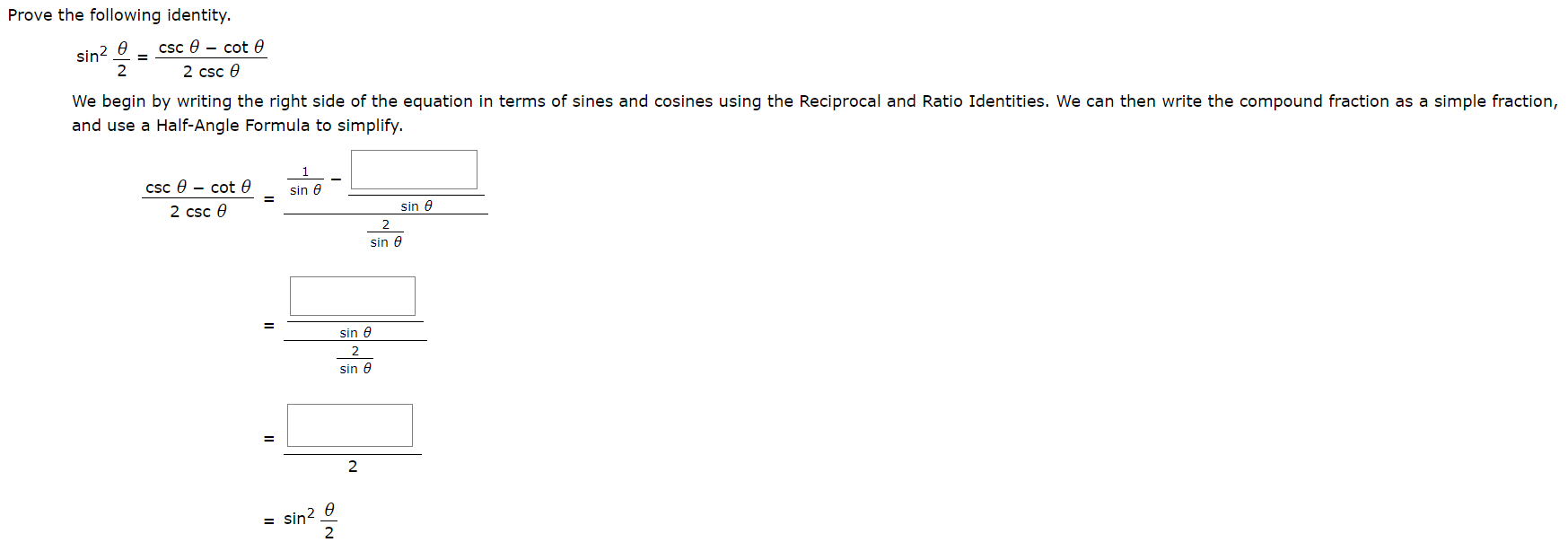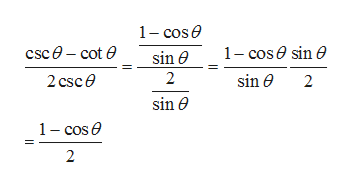# Prove the following identity.csc cot esin222 cscWe begin by writing the right side of the equation in terms of sines and cosines using the Reciprocal and Ratio Identities. We can then write the compound fraction as a simple fraction,and use a Half-Angle Formula to simplify.csc cot esin esin2 csc e2sin esin e2.sin e2esin2

Question

photo attachedhelp_outlineImage TranscriptioncloseProve the following identity. csc cot e sin2 2 2 csc We begin by writing the right side of the equation in terms of sines and cosines using the Reciprocal and Ratio Identities. We can then write the compound fraction as a simple fraction, and use a Half-Angle Formula to simplify. csc cot e sin e sin 2 csc e 2 sin e sin e 2. sin e 2 e sin2 fullscreen
check_circleExpert Solution
Step 1

We begin by writing right hand side of equation in terms of sines and cosines by using reciprocal and ratio identities

Step 2

On simplifying ...help_outlineImage Transcriptionclose1- cose csc0-cot e 1-cose sin e sin 2 sin e 2 csce 2 sin e 1 cos e 2 fullscreen

### Want to see the full answer?

See Solution

#### Want to see this answer and more?

Solutions are written by subject experts who are available 24/7. Questions are typically answered within 1 hour*

See Solution
*Response times may vary by subject and question
Tagged in

### Trigonometric Ratios